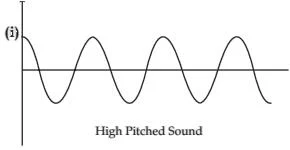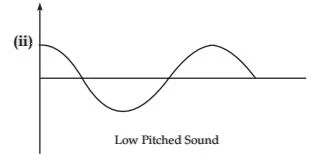## Extra Questions for Class 9th: Ch 12 Sound (Science) Important Questions Answer Included

Very Short Answer Questions (VSAQs): 1 Mark

Q1. What is a supersonic speed?
When the speed of an object exceeds the speed of sound, it is said to travel in supersonic speed. For example, bullets, jet crafts etc., travel in supersonic speeds.

Q2. Which type of wave is a sound wave?
Longitudinal mechanical wave.

Q3. Why is sound wave called a longitudinal wave?
In a sound wave, the particles of the medium vibrate in the direction parallel to the direction of the propagation of disturbance. Hence, a sound wave is called a longitudinal wave.

Q4. A baby recognizes her mother by her voice. Name the characteristic of sound involved.
Quality or timbre

Q5. Which sound has higher pitch-guitar or car horn?
The frequency of the vibration of a sound produced by a guitar is greater than that produced by a car horn. Since the pitch of a sound is proportional to its frequency, the guitar has a higher pitch than a car-horn.

Short Answer Questions-I (SAQs-I) : 2 Marks

Q1. (i) Define the time period of a wave.
(ii) Give the relation among speed of sound v, wavelength λ and its frequency υ.
(iii) A sound wave travels at a speed of 339ms-1. If its wavelength is 1.5 cm, what is the frequency of the wave ?

(i) The time taken by two consecutive compressions or rarefactions to cross a fixed point is called the time period of a wave.

(ii) Speed of sound (v)=Wavelength(λ)×Frequency (u)
⇒ v = λu

(iii) Given, velocity, v = 339ms-1
Wavelength, λ=1.5 cm
= 1.5/100 m
We know,
Velocity = Wavelength × Frequency
Therefore, Frequency = Velocity/Wavelength= 339/(1.5/100)
= (339×100)/1.5
Frequency = 22600 Hz

Q2. (a) Write the factor on which pitch of a sound depends?
(b) Draw a diagram to represent sound of:
(i) High pitch
(ii) Low pitch, of the same loudness.

(a) The pitch of a sound depends on the frequency of vibration. The pitch of a sound is directly proportional to its frequency.

(b)Q3. (a) Which wave property determines:
(i) Loudness,
(ii) Pitch.
(b) Why are the ceilings of concert halls curved?

(a) (i) Amplitude
(ii) Frequency.

(b) Ceiling of concert halls are curved so that sound, after reflection, reaches all the corners of the hall. The sound after reflection from the curved surface spreads evenly across the width of the hall.

Q4. A wave is moving in air with a velocity of 340 m/s. Calculate the wavelength if its frequency is 512 Hz.

Frequency = 512 Hz, Speed of the sound = 340 m/s
Since v= λ u
λ = v/u = 340/512 = 0.66m

Q5. A stone is thrown in a pond. 12 full ripples are produced in 1 second. If the distance between a crest and a trough is 10 cm, calculate the wavelength and velocity of the wave.

Given :
Distance between the crest and next trough = 10 cm
or λ/2=10
λ=20 cm=0.20 m
Velocity v=u × λ
=12 ×0.20
=2.40 m/s

Short Answer Questions-II (SAQs-II) : 3 Marks

Q1. When vertically jerk is given to a string, transverse waves are formed. Give three features of these waves.

Three features of transverse waves are:
(i) The particles of the medium vibrates at right angles to the direction of propagation of wave.
(ii) Transverse waves travels in the form of crests and troughs.
(iii) They cannot travel through vacuum.

Q2. List any three human activities that would lead to environmental pollution.

(i) Burning of wood.
(ii) Combustion of coal and petroleum fuels.
(iii) Use of chemicals in the form of fertilizers and pesticides.

Q3. (a) Name the type of waves that can travel in gases.
(b) Name the wave which can travel in solids, liquids as well as gas.
(c) At any instant, a compression is formed at a point. After how much time period
(i) a rarefaction,
(ii) a compression will be formed at the same point ?

(a) Longitudinal wave.
(b) Sound waves.
(c) If T is the time period of the wave then,
(i) After time interval T/2, the point will have a rarefaction
(ii) After time T, the point will have a compression.

Q4. (i) How are the wavelength and frequency of a sound wave related to its speed ?
(ii) Calculate the wavelength of a sound wave whose frequency is 220 Hz and speed is 440 m/s in a given medium.

(i) v = λf
(ii) λ = v/f= 440/220 = 2 m

Q5. When a stone is thrown into a pond what type of waves are produced? Draw its displacement time graph. Label crest, trough and wavelength on the graph.

When a stone is thrown into a pond, transverse waves are formed.Long Answer Questions (LAQs) : 5 Marks

Q1. (a) Why are sound waves called mechanical waves? List two practical applications of reflection of these waves.
(b) A stone is dropped from the top of a tower 125 m high into a pond of water at the base of the tower. When is the splash heard at the top ? (𝒈=𝟏𝟎𝒎/𝒔2 𝒂𝒏𝒅 𝒔𝒑𝒆𝒆𝒅 𝒐𝒇𝒔𝒐𝒖𝒏𝒅 =𝟑𝟒𝟎𝒎/𝒔)

(a) Sound is produced by the vibration of objects. When objects vibrate, it collides with the molecules in the medium. This collision produces mechanical oscillations in the medium. Because of this, sound waves are known as mechanical waves.
(i) Megaphones, horns, musical instruments as trumpets etc.
(ii) Stethoscope.

(𝑏)𝑆𝑝𝑒𝑒𝑑 𝑜𝑓 𝑠𝑜𝑢𝑛𝑑=340𝑚/𝑠;
𝑔 = 10 𝑚/𝑠2;
𝑢 = 0;
ℎ = 125 𝑚;
𝑡 = ?
ℎ = 𝑢𝑡+ 1/2 𝑔𝑡2
125 = 0 + 1/2×10×𝑡2
∴𝑡 = 5𝑠
Let 𝑡1 be the time taken by the sound to reach top
𝑢 = 340 𝑚/𝑠,
ℎ = 125 𝑚, 𝑡1=?
𝑡1 = ℎ/𝑢 = 125/240 = 0.37𝑠
𝑆𝑝𝑙𝑎𝑠ℎ 𝑤𝑖𝑙𝑙 𝑏𝑒 ℎ𝑒𝑎𝑟𝑑 𝑎𝑓𝑡𝑒𝑟 𝑡+ 𝑡1 = (5+0.37)𝑠 = 5.37𝑠

Q2. A sound wave travelling in a medium is represented as shown in the figure:
(i) Which letter represents the amplitude of the sound wave?
(ii) Which letter represents the wavelength of the wave?
(iii) What is the frequency of the source of sound if the vibrating source of sound makes 360 oscillations in two minutes?
(iv) Calculate the time period of the source of sound.